# Python:线性代数-机器学习背后的优化原理 (五十五)

## 准备工作

Latex cheatsheet

aTeX Cookbook

# TODO: import相关库
import numpy as np
import pandas as pd
import matplotlib.pyplot as plt
import seaborn as sb

# 使用 notebook 中的魔法指令 %matplotlib inline，否则会导致你接下来无法打印出图像
%matplotlib inline

## 1、标量，向量，矩阵，张量

• 标量（scalar）：形式而言，一个标量是一个单独的数，常用斜体的小写变量名称来表示。v

• 向量（vector）：形式而言，一个向量是一列有序数，常用粗体的小写变量名称表示v，或者上面标记剪头$\vec{v}$

• 矩阵（matrix）：形式而言，一个矩阵是一个二维数组，常用大写变量名称表示A，表示内部的元素则会使用$A_{i,j}$

• 张量（tensor）：形式而言，一个张量是一个多维数组，常用粗体的大写字母变量名称表示T，表示内部的元素则会使用$A_{i,j,z}$ 等等• 加法• 标量乘法• 转置• 矩阵向量乘法（内积，人工智能中常见的拼写：matrix product 或者 dot product）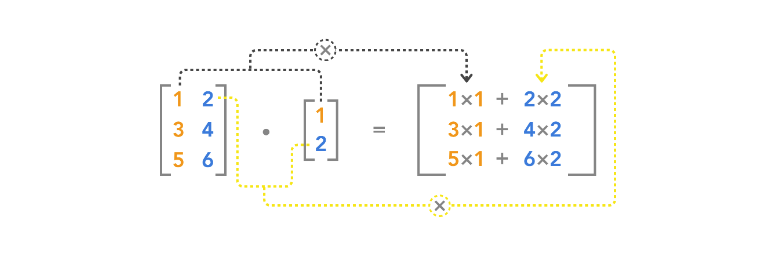$$\begin{bmatrix} A{1,1} & A{1,2} & \cdots & A{1,n} \\ A {2,1} & A{2,2} & \cdots & A{2,n} \\ \cdots & \cdots & \cdots & \cdots \\ A{m,1} & A{m,2} & \cdots & A_{m,n} \end{bmatrix} \times \begin{bmatrix} x_1 \\ x_2 \\ \cdots \\ x_n \end{bmatrix} \begin{bmatrix} b_1 \\ b_2 \\ \cdots \\ b_m \end{bmatrix}$$

$$Ax=b$$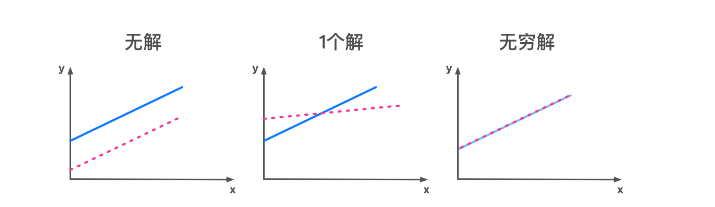### 1.1、基本运算并绘图

$\hspace{1cm}\vec{v} = \begin{bmatrix} 1\ 1\end{bmatrix}$

$\hspace{1cm}\vec{w} = \begin{bmatrix} -2\ 2\end{bmatrix}$

$$\hspace{1cm}\vec{v}+\vec{w} = \begin{bmatrix} -1\ 3\end{bmatrix}$$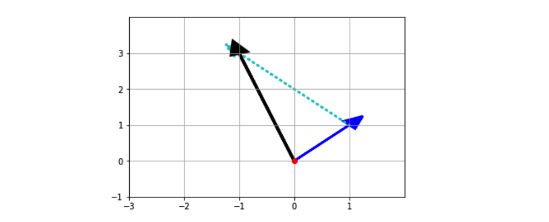#### 1.1.1

$$\hspace{1cm}\vec{v} = \begin{bmatrix} 4\ 1\end{bmatrix}$$

$$\hspace{1cm}\vec{w} = \begin{bmatrix} -1\ 2\end{bmatrix}$$

# 1.1.1 TODO：
v = np.array([4, 1])
vv = 2 * v
w = np.array([-1, 2])
res = vv - w

# Creates axes of plot referenced 'ax'
ax = plt.axes()

# Plots red dot at origin (0,0)
ax.plot(0,0,'or')

# Plots vector v as blue arrow starting at origin 0,0
ax.arrow(0, 0, *v, color='b', linewidth=2.5, head_width=0.30, head_length=0.35)
ax.arrow(res, res, *w, color='c', linewidth=2.5, head_width=0.30, head_length=0.35)

# Plots vector vv as green arrow starting at origin 0,0
ax.arrow(0, 0, *vv , color='g', linewidth=2.5, head_width=0.30, head_length=0.35)
ax.arrow(0, 0, *res, color='r', linewidth=2.5, head_width=0.30, head_length=0.35,  linestyle='dotted',)

# 设置 x 坐标
plt.xlim(-1,12)

major_xticks = np.arange(-1, 12)
ax.set_xticks(major_xticks)

# 设置 y 轴坐标
plt.ylim(-1, 4)
major_yticks = np.arange(-1, 4)
ax.set_yticks(major_yticks)
plt.grid(b=True, which='major')

# Displays final plot
plt.show()$$\begin{cases} y = 2x + 1\\ y = 6x - 2 \end{cases}$$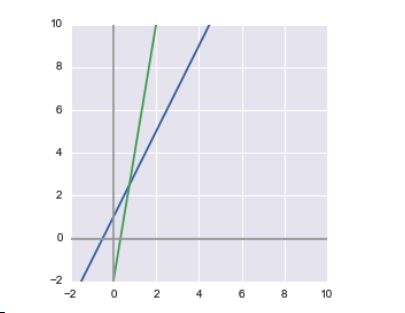$$\begin{cases} x = \frac{3}{4} \\ y = \frac{5}{2} \end{cases}$$

#### 1.1.2

$$\begin{cases} y = 2x + 1\\ y = \frac{1}{10}x+6 \end{cases}$$

# 1.1.2 TODO

def check_vector_span(set_of_vectors, vector_to_check):
# Creates an empty vector of correct size
vector_of_scalars = np.asarray([None]*set_of_vectors.shape)

# Solves for the scalars that make the equation true if vector is within the span
try:
# Use np.linalg.solve() function here to solve for vector_of_scalars
vector_of_scalars = np.linalg.solve(set_of_vectors, vector_to_check)
if not (vector_of_scalars is None):
print("\nVector is within span.\nScalars in s:", vector_of_scalars)

# Handles the cases when the vector is NOT within the span
except Exception as exception_type:
if str(exception_type) == "Singular matrix":
print("\nNo single solution\nVector is NOT within span")
else:
print("\nUnexpected Exception Error:", exception_type)
return vector_of_scalars

vw = np.array([[2,-1],[1/10,-1]])
t = np.array([-1, -6])

print("\nMatrix vw:", vw, "\nVector t:", t, sep="\n")

s = check_vector_span(vw,t)

# 两点确定一条直线
x = np.arange(0,10,0.1)
y1 = 2*x + 1
y2 = 0.1*x + 6
plt.plot(x, y1,'b',linewidth=3)
plt.plot(x, y2,'c-.',linewidth=3)
plt.plot(s,s,'ro',linewidth=3)
plt.xlabel('Single Solution')
plt.text(6, 14.5, 'y=2x+1',fontsize = 15, rotation = 30)
plt.text(6, 5, 'y=0.1x+6',fontsize = 15, rotation = 0)
plt.show()

Matrix vw:
[[ 2.  -1. ]
[ 0.1 -1. ]]

Vector t:
[-1 -6]

Vector is within span.
Scalars in s: [2.63157895 6.26315789]# 由上图可知，方程组有唯一解

### 1.2、说明题

#### 1.2.1

$$A=\begin{bmatrix} 21 & 7 \\ 15 & 42 \\ 9 & 6 \end{bmatrix}, B=\begin{bmatrix} 4 \\ 33 \end{bmatrix}$$

# 1.2.1 TODO
A = np.array([[21,7],[15,42],[9,6]])
B = np.array([,])

AB = np.matmul(A ,B)
ABT = AB.T

BT = B.T
AT = A.T

BTAT = np.matmul(BT, AT)
print(ABT)
print(BTAT)

print(ABT==BTAT)
[[ 315 1446  234]]
[[ 315 1446  234]]
[[ True  True  True]]

#### 1.2.2

$$A=\begin{bmatrix} 9 & 3 \\ 8 & 4 \\ 7 & 6 \end{bmatrix}, B=\begin{bmatrix} 5 \\ 2 \end{bmatrix}, C=\begin{bmatrix} 5 \\ 7 \end{bmatrix}$$

# 1.2.2 TODO
A = np.array([[9,3],[8,4],[7,6]])
B = np.array([,])
C = np.array([,])

# ABAC = A * (B + C)
ABAC = np.matmul(A, B+C)
print(ABAC)

AB = np.matmul(A, B)
AC = np.matmul(A, C)

print(AB)
print(AC)

ABAC_PLUS = AB + AC
print(ABAC_PLUS)

print(ABAC == ABAC_PLUS)

[

]
[

]
[

]
[

]
[[ True]
[ True]
[ True]]

## 2、特殊矩阵

• 单位矩阵

• 逆矩阵

• 对角矩阵

• 对称矩阵

• 正交矩阵

### 2.1、证明题

$A^{-1} A = I_n$
$A^{-1} = I_n / A$
$x = A^{-1}b = I_n / A b$
$A x = A (I_n / A b) = A I_n / A b = I_n b = b$

### 2.2、 计算题

#### 2.2.1

$$\begin{cases} y = 2x + 1\\ y = \frac{1}{10}x+6 \end{cases}$$

# 2.2.1 TODO
# A = np.array([[2,-1],[1/10,-1]])  # 多维数组
# t = np.mat('2 -1;0.1 -1')  # 这种写法也可以
t = np.mat([[2,-1],[0.1,-1]])
# type(t) # 打印：numpy.matrixlib.defmatrix.matrix
print(t)
# b = np.array([-1, -6]) # numpy.ndarray  数组,数组类型如果使用b.I求逆矩阵会报错
# b = np.mat('-1;-6')
b = np.mat([[-1],[-6]])
# print(b)

# 通过逆矩阵求得答案
t.I*b

[[ 2.  -1. ]
[ 0.1 -1. ]]

matrix([[2.63157895],
[6.26315789]])
# 通过方法求解
np.linalg.solve(t,b)

# 由以上返回的结果可得，两者相等
matrix([[2.63157895],
[6.26315789]])
# 逆矩阵
t.I
matrix([[ 0.52631579, -0.52631579],
[ 0.05263158, -1.05263158]])
# t * t.I(矩阵乘以逆矩阵)
R = np.matmul(t, t.I)
print(R)
[[1. 0.]
[0. 1.]]

#### 2.2.2

1、请用numpy（或自写算法）实现一个6x6的对角矩阵，矩阵的对角线由3至8（含8）组成。

2、计算第一问生成的对角矩阵与向量$[6,7,1,2,5,9]^{T}$的乘积

# 2.2.2 TODO
x = np.arange(3,9)
# print(x)

# 单位矩阵是主对角线上全是 1，其他位置全是 0 的方形矩阵。
n = np.eye(6)

diag6 = x * n
print(diag6)
#diag=np.diag(x)

[[3. 0. 0. 0. 0. 0.]
[0. 4. 0. 0. 0. 0.]
[0. 0. 5. 0. 0. 0.]
[0. 0. 0. 6. 0. 0.]
[0. 0. 0. 0. 7. 0.]
[0. 0. 0. 0. 0. 8.]]
v = np.array([6,7,1,2,5,9])
# print vt
VT = v.T
MT = np.matmul(diag6,VT)
print(MT)
[18. 28.  5. 12. 35. 72.]

## 3、迹运算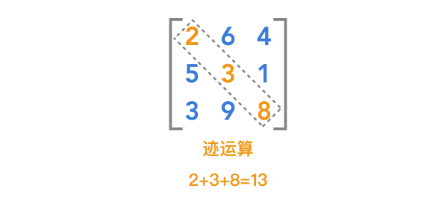$$\large Tr(A) = \sum{i}A{i,i}$$

$$\large Tr(ABC) = Tr(CAB) = Tr(BCA)$$

$$A= \begin{bmatrix} 7 & 6 \\ 29 & 3 \end{bmatrix}$$

$$B= \begin{bmatrix} 2 & -8 \\ 9 & 10 \end{bmatrix}$$

$$C= \begin{bmatrix} 2 & 17 \\ 1 & 5 \end{bmatrix}$$

# 3 TODO
A = np.array([[7,6],[29,3]])
B = np.array([[2,-8],[9,10]])
C = np.array([[2,17],[1,5]])

AB = np.matmul(A,B)
ABC = np.matmul(AB,C)

print(np.diag(ABC))
diagABC = np.diag(ABC).sum()
print(diagABC)

[140 435]
575
CA = np.matmul(C,A)
CAB = np.matmul(CA,B)
diagCAB = np.diag(CAB).sum()

BC = np.matmul(B,C)
BCA = np.matmul(BC,A)
diagBCA = np.diag(BCA).sum()
print(diagABC,diagCAB,diagBCA)

575 575 575

## 4、衡量向量以及矩阵的大小：范数与条件数

### 范数的定义

$$\large {\Vert x \Vert}{p} = \lgroup {\sum{i}{\vert x_i \vert}^p }\rgroup ^{\frac{1}{p}}$$

L0 范数
$$\large \Vert x \Vert = \sqrt{\sum_i x_i^0} = #(i|xi \neq0)$$
L1 范数
$$\large {\Vert x \Vert} {1} = \lgroup {\sum_{i}{\vert xi \vert} }\rgroup$$
L2 范数
$$\large {\Vert x \Vert} {2} = \lgroup {\sum_{i}{\vert x_i \vert}^2 }\rgroup ^{\frac{1}{2}}$$

$$\large {\Vert x \Vert}^{\infty} = \max_{i}{\vert x_i \vert}$$

### 4.1、计算向量的范数

# TODO 实现这里向量范数计算的函数，要求可以计算p = 0,1,2,3 ... 无穷 情况下的范数

""" 计算向量的范数
参数
x: 向量 numpy数组 或者list数组
p: 范数的阶，int型整数或者None
infty: 是否计算max范数，bool型变量，True的时候表示计算max范数，False的时候计算p范数

返回
向量的范数，float类型数值

hint:
1.你需要首先判断infty是True or False, 然后判断p 是否为零
2.注意int类型变量在计算时候需要规整为float类型

"""
def calc_Norm(x, p = 2, infty = False):
if infty == False:   #计算p范数
if p == 0:
return np.nonzero(x).size #非零元素个数
else:
# 使用 np.abs()将x中的元素取其绝对值，内置函数power，仅用于单个元素的次幂运算
return np.power(np.power(np.abs(x),p).sum(),1/p)

else:
return np.max(x) # x最大数
%run -i -e test.py LinearRegressionTestCase.test_calc_Norm
.
----------------------------------------------------------------------
Ran 1 test in 0.039s

OK

<Figure size 432x288 with 0 Axes>

### 4.2、计算矩阵的范数

$$\large {\Vert A \Vert}{F} = \sqrt {\sum{i,j}{\vert A_{i,j} \vert}^2 }$$

# TODO 实现这里矩阵Frobenius范数计算的函数

""" 计算向量的范数
参数
A: 给定的任意二维矩阵 list或者numpy数组形式

返回
矩阵的Frobenius范数，float类型数值

"""
def calc_Frobenius_Norm(A):
return np.power(np.power(np.abs(A),2).sum(), 1/2)
%run -i -e test.py LinearRegressionTestCase.test_calc_Frobenius_Norm
.
----------------------------------------------------------------------
Ran 1 test in 0.024s

OK

### 4.3、计算矩阵的条件数

$$\large \kappa{(A)} = {\Vert A \Vert}_F {\Vert A^{-1} \Vert}_F$$

""" 计算矩阵的条件数
参数
A: 给定的任意二维矩阵 list或者numpy数组形式

返回
矩阵的condition number,

"""
def calc_Condition_Number(A):
if np.linalg.det(A) != 0:
# np.linalg.inv()：矩阵求逆
# np.linalg.det()：矩阵求行列式（标量）
return calc_Frobenius_Norm(A)*calc_Frobenius_Norm(np.linalg.inv(A))
else:
return float('inf')
%run -i -e test.py LinearRegressionTestCase.test_calc_Condition_Number
.
----------------------------------------------------------------------
Ran 1 test in 0.034s

OK

### (选做)4.4、条件数的理解与应用

a. 有如下两个2*2的非奇异矩阵A和B:

$A = \begin{bmatrix} 1 &2 \ 3 &4 \ \end{bmatrix}$

$B = \begin{bmatrix} 1 &2 \ 2 &4.0001 \ \end{bmatrix}$

b. 根据上面构造的矩阵A,B分别计算线性系统方程组的解$w$:

A $\begin{bmatrix}w{a1}\w{a2}\ \end{bmatrix}$ = $\begin{bmatrix}1\2\ \end{bmatrix}$,

B $\begin{bmatrix}w{b1}\w{b2}\ \end{bmatrix}$ = $\begin{bmatrix}1\2\ \end{bmatrix}$,

A $\begin{bmatrix}w{a1}\w{a2}\ \end{bmatrix}$ = $\begin{bmatrix}{1.0001}\{2.0001}\ \end{bmatrix}$,

B $\begin{bmatrix}w{b1}\w{b2}\ \end{bmatrix}$ = $\begin{bmatrix}{1.0001}\{2.0001}\ \end{bmatrix}$.

c. 计算完成之后，比较condition number大小与线性系统稳定性之间的关系，并且给出规律性的总结；

d. 阅读与思考: 考虑更为通用的一种情况，我们计算一个典型的线性回归系统:

$$Xw = b$$

1.等式两边乘以$X^T$
$$X^TXw = X^Tb$$
2.等式两边乘以$(X^TX)^{-1}$
$$(X^TX)^{-1}X^TXw = (X^TX)^{−1}X^Tb$$
3.因为$A^{-1}A = I$，两边约去即可得：
$$w=(X^TX)^{−1}X^Tb$$

hint1. 单位矩阵的condition number是最低的，是最为稳定的；

hint2. 如果要使得该系统存在闭式解，那么就必须使得求逆运算是可以进行的，也就是说重新构造的$X^TX$必须是可逆的方阵；

hint3. 重新构造的方式可以是在$X^TX$基础上进行加或者减或者乘除相关矩阵的操作；

$$w = (X^TX+\lambda I)^{−1}X^Tb$$

$$J_w = min({\Vert Xw -y \Vert}^2 + \alpha {\Vert w \Vert}^2)$$

$${\Vert Xw -y \Vert}^2 + \alpha {\Vert w \Vert}^2 = (Xw -y)^T (Xw-y) + \alpha w^Tw$$

$$X^T (Xw-y) + \alpha w = 0$$

$$w = (X^TX+\lambda I)^{−1}X^Tb$$

## 5、SVD

SVD是Singular value decomposition的缩写，称为奇异值分解，是分解矩阵的一种方式，会将矩阵分解为奇异向量（singular vector）和奇异值（singular value），分解的意义其实很明确，就是想将一个很大很复杂的矩阵，用更小更简单的几个子矩阵的相乘来表示，这些小矩阵描述的是矩阵的重要的特性。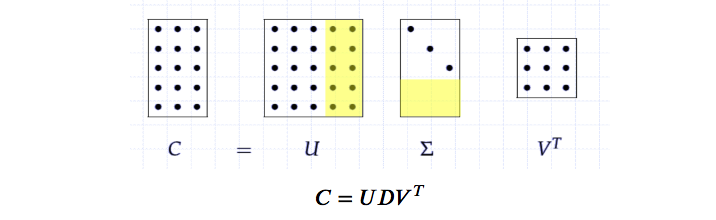$$\large C = UDV^{T}$$

SVD最常用的做法就是用来进行特征的降维以及矩阵的低秩重构，例如这里分别取矩阵U、D、VT的前k列，如图示中的白色部分，然后重新计算新的C矩阵，即为k维度下的矩阵重构，这种方法被广泛应用于自然语言处理LSA、推荐系统SVD++,FM,FFM等领域，如有兴趣可以继续参考链接相关资料。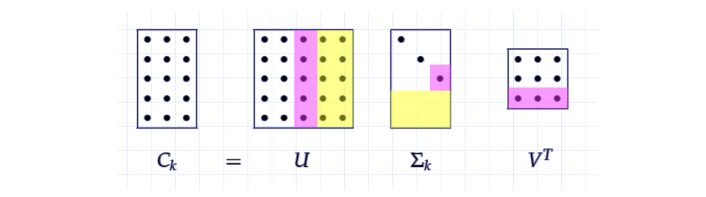### 5.1、使用numpy去计算任意矩阵的奇异值分解：

""" 计算任意矩阵的奇异值分解
参数
A: 给定的任意二维矩阵 list或者numpy数组形式

返回
使用numpy.linalg相关函数，直接返回分解之后的矩阵U,D,V
（可以尝试一下使用np.shape一下分解出来的U，D，VT，会发现维度跟我们上面讲解所描述的不同，
暂时不用管他直接返回np求解出的U，D，VT即可）

"""
def calc_svd(A):
U, D, V = np.linalg.svd(A)
return U, D, V

%run -i -e test.py LinearRegressionTestCase.test_calc_svd
.
----------------------------------------------------------------------
Ran 1 test in 0.708s

OK

<Figure size 432x288 with 0 Axes>

### (选做) 5.2、利用奇异值分解对矩阵进行降维

# TODO 利用SVD进行对于矩阵进行降维

""" 利用SVD进行对于矩阵进行降维
参数
A: 给定的任意二维矩阵 list或者numpy数组形式 shape为(m,n)
topk: 降维的维度 (m,n) -> (m,topk)

返回
降维后的矩阵 (m, topk)

hint
1. 对角矩阵D存在一个较为明显的特性，就是D的对角线元素是递减的，这些元素实际上是衡量了所分解的矩阵U,V的列向量的重要性
2. 因此我们常说的svd降维就是利用选取的前topk大的对角线矩阵元素进行构造新的降维矩阵
3. U的按照前topk截取的列向量 * topk截取的对角矩阵 即为新的降维后的矩阵

"""
def calc_svd_decompostion(A, topk = 2):
U, D, V = np.linalg.svd(A)
return np.matmul(U[:,:topk],(D*np.eye(len(D)))[:topk,:topk])
%run -i -e test.py LinearRegressionTestCase.test_calc_svd_decompostion

### (选做) 5.3、利用奇异值分解对矩阵进行降维后重构

""" 利用SVD进行对于矩阵进行降维
参数
A: 给定的任意二维矩阵 list或者numpy数组形式 shape为(m,n)
topk: 降维的维度 (m,n) -> (m,topk)

返回
降维重构后的矩阵 (m, n)
hint
这里除了降维矩阵外，另外一个较为常见的应用就是对矩阵进行重构，具体的做法类似前面的思路
1. 选取对应的U，D，V的topk向量
2. U的按照前topk截取的列向量 * topk截取的对角矩阵 * V^T按照前topk截取的行向量(注意这里是V的转置,因为分解得到的是V^T)

"""
def calc_svd_reconsitution(A, topk = 2):
U, D, V = np.linalg.svd(A)
return np.matmul(calc_svd_decompostion(A, topk),V[:topk,:])
%run -i -e test.py LinearRegressionTestCase.test_calc_svd_reconsitution
.
----------------------------------------------------------------------
Ran 1 test in 0.316s

OK

### (选做) 5.4、计算不同降维大小重构矩阵的Frobenius范数损失

$$\Large Loss_{F} = {\Vert A - A_k \Vert}_F$$

## 不要修改这里！
import numpy as np
from sklearn.datasets import load_boston
import matplotlib.pyplot as plt
%matplotlib inline
A = load_boston()['data']  # 载入boston house 数据集
print(A.shape)
(506, 13)
loss_hist = []
for topk in range(1,13):
# 5.4 TODO
### 1.计算相应的SVD topk降维后的重构矩阵，需实现calc_svd_reconsitution
Ak = calc_svd_reconsitution(A, topk)
### 2.计算对应的F范数损失，并存储loss放入loss_hist列表中
loss = calc_Frobenius_Norm(A-Ak)
loss_hist.append(loss)

### 画出F损失随着降维大小的变化图
### x坐标为对应的降维大小，y坐标为对应的F损失
plt.plot(range(1,13),loss_hist,'r--')
plt.xlabel('decomposition size')
plt.ylabel('F Loss')
Text(0,0.5,'F Loss')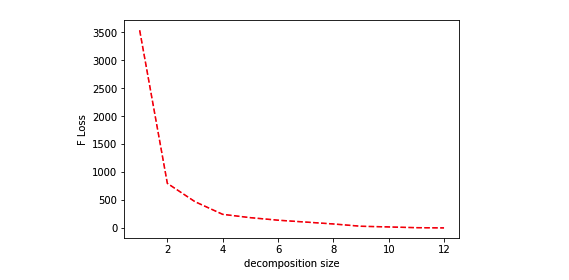### 5.5、SVD的有趣应用

• 需要使用 PIL 加载你所选择的图像（文档）,所以记得导入需要的包（模块）
• 使用Image的convert方法将图像变为灰度图
• 将convert后的结果转换成np.array,需用到Image.getdata方法来读取图片每个pixel的数据，特别注意一下，对于彩色的图来说，即使我们转为了灰度图，但每一个pixel还是由RGB三个维度组成，所以在getdata时，band需要设定为某一个颜色index，比如band = 0，这样只用R这个维度的数据。用这个方法来保证图片的每个pixel只占有一个单元的空间。
• 因为我们转np.array时破坏了原有图形的样子，变成了一个一维数据，我们要将转换后的np.array恢复到图片应有的size，转换后，可以shape确认下是否与最开始转出的灰度图的size一致，注意图的size是（宽，高），而宽对应array.shape的应该是列，而高对应的是行，别弄反了。
• 使用上方实现的calc_svd函数计算上一步计算出的np.array数据，赋值给变量：U,D,VT
• 打印出U,D,VT的shape形状，尤其注意观察D的shape
• 在U，VT，D变量成功实现的情况下，运行测试程序看效果
# 5.5 TODO
#请在U，D，V变量完成的情况下调用此测试程序，不要修改此处
plt.figure(figsize=(16,6))
for i,topk in enumerate([5, 10, 15, 20, 30, 50]):
reconstimg = np.matrix(U[:, :topk]) * np.diag(D[:topk]) * np.matrix(VT[:topk, :])
plt.subplot(231+i)
plt.imshow(reconstimg, cmap='gray')
title = "n = %s" % ((i+1)*5)
plt.title(title)
plt.show()